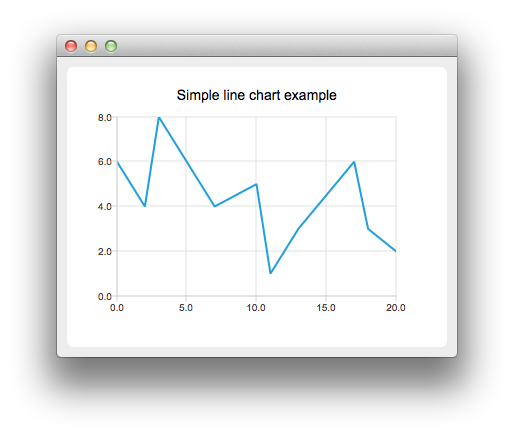# Creating Line Charts

Note: This is part of the Charts with Widgets Gallery example.To create a line chart, a QLineSeries instance is needed. Let's create one.

`auto series = new QLineSeries;`

Then we add data to the series. We can use the append() member function or use the stream operator.

```series->append(0, 6);
series->append(2, 4);
series->append(3, 8);
series->append(7, 4);
series->append(10, 5);
*series << QPointF(11, 1) << QPointF(13, 3) << QPointF(17, 6) << QPointF(18, 3) << QPointF(20, 2);```

To present the data on the chart we need a QChart instance. We add the series to it, create the default axes, and set the title of the chart.

```auto chart = new QChart;
chart->legend()->hide();
`createDefaultChartView(chart);`